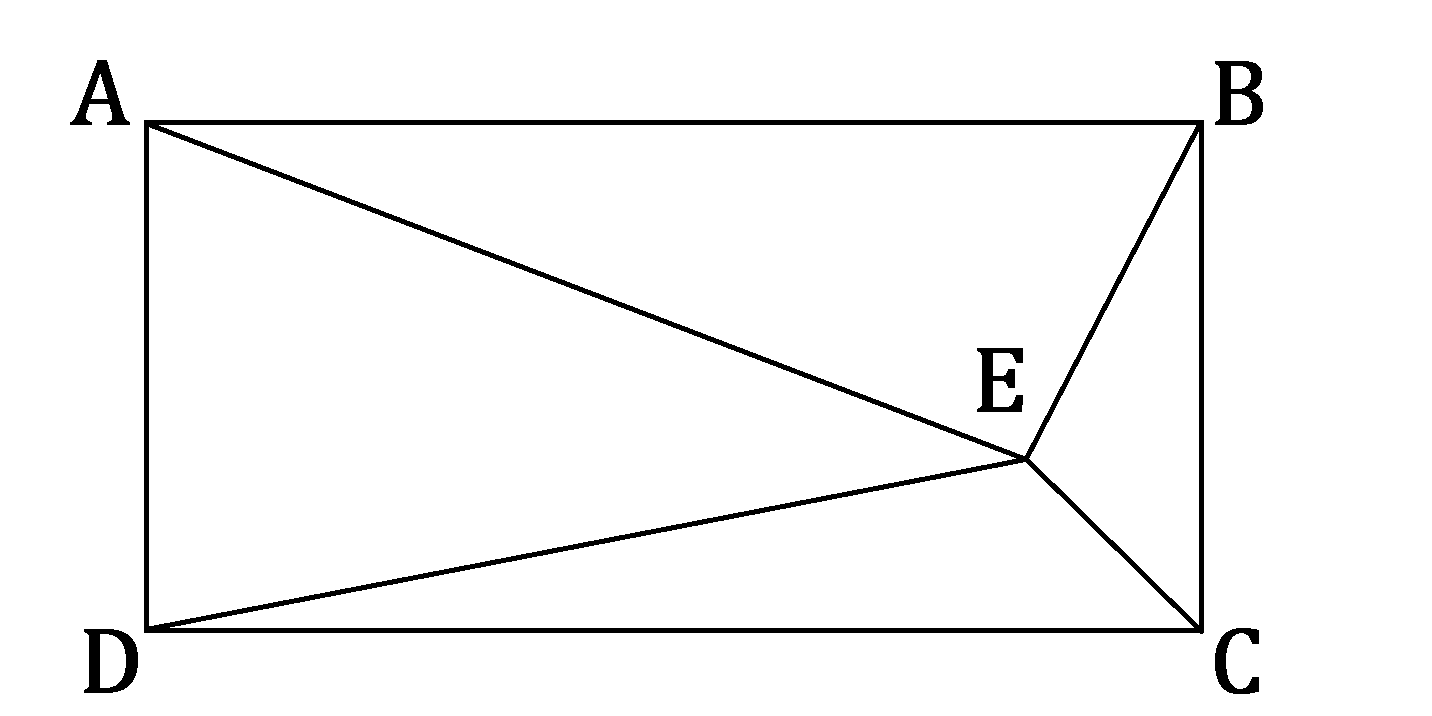# Mystery Spot

Point $E$ lies within rectangle $ABCD,$ as shown below.

If the distances from the vertices to point $E$ are all distinct integers, what is the least possible value of $AE + BE + CE + DE?$×